# Class 8 Maths NCERT Solutions for Chapter – 13 Direct and Inverse Proportions Ex – 13.2

## Direct and Inverse Proportions

Question 1.
Which of the following are in inverse proportion?
(i)
The number of workers on a joh and the time to complete the job.
(ii) The time taken for a journey and the distance travelled in a uniform speed.
(iii) Area of cultivated land and the crop harvested.
(iv) The time taken for a fixed journey and the speed of the vehicle.
(v) The population of a country and the area of land per person.

Solution:
(i) We know that more is the number of workers to do a job, less is the time taken to finish the job.
So, it is a case of inverse proportion.
(ii) Clearly, more is the time taken, more is the distance travelled in a uniform speed.
So, it is a case of direct proportion.
(iii) Clearly, more is the area cultivated land, more is the crop harvested. So, it is a case of direct proportion.
(iv) We know that more is the speed of the vehicle, less is the time to cover a fixed distance.
So, it is a case of inverse proportion.
(v) Clearly, more is the population, less is the area of land per person in a country.
So, it is a case of inverse proportion.

Question 2.
In a Television game show, the prize money of ₹ 1,00,000 is to be divided equally amongst the winners. Complete the following table and find whether the prize money given to an individual winner is directly or inversely proportional to the number of winners?

Solution:
Clearly, more the number of winners, less is the prize for each winner. So, it
∴ 4 x x = 1 x 100000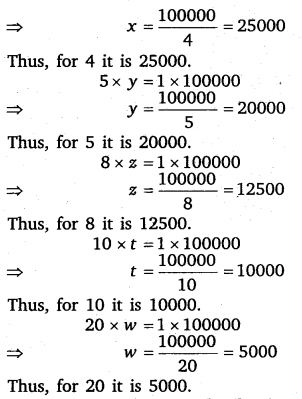Question 3.
Rehman is making a wheel using spokes. He wants to fix equal spokes in such a way that the angles between any pair of consective spokes are equal. Help him by completing the following table.(i) Are the number of spokes and the angles formed between the pairs of consecutive spokes in inverse proportion?
(ii) Calculate the angle between a pair of consecutive spokes on a wheel with 15 spokes.
(iii) How many spokes would be needed, if the angle between a pair of consecutive spokes is 40°?

Solution:
Clearly, more is the number of spokes, less is the measure of angle between a pair of consecutive spokes.
Also, we observe here
4 x 90° = 6 x 60° (= 360°)
So, it is a case of inverse proportion.(i) Yes, the number of spokes and the angles formed between the pairs of consecutive spokes are in inverse proportion.
(ii) Let x° be the angle between a pair of consecutive spokes on a wheel with 15 spokes.
∴ 15 x x = 4 x 90 ⇒ x=$\frac { 4\times 90 }{ 15 }$ = 24
Thus, the required angle is 24°.
(iii) Let x spokes are needed on a wheel if the angle between a pair of consecutive spokes is 40°.
∴ x x 40 = 4 x 90 ⇒ x=$\frac { 4\times 90 }{ 40 }$ = 9
Thus, the required number of spokes is 9.

Question 4.
If a box of sweets is divided among 24 children, they will get 5 sweets each. How many would each get, if the number of the children is reduced by 4?

Solution:
Since the number of the children were reduced by 4. Therefore, their number becomes 24 – 4 = 20.
Let each child get x sweets when their number is 20.
Thus, we have the following table :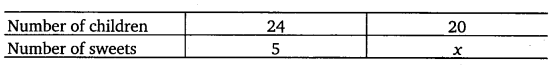Since less children will get more sweets. So, it is a case of inverse proportion.
∴ 24 x 5 = 20 x x ⇒ x=$\frac { 24\times 5 }{ 20 }$ = 6
Hence, each child will get 6 sweets.

Question 5.
A farmer has enough food to feed 20 animals in his cattle for 6 days. How long would the food last if there were 10 more animals in his cattle?

Solution:
Let the food will now last for x days. Then,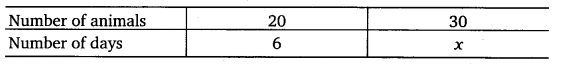Clearly, more is the number of animals, less will be the number of days for food to last. .
So, it is a case of inverse proportion.
∴ 24 x 6 = 30 x x ⇒ x=$\frac { 20\times 6 }{ 30 }$ = 4
Hence, the food will now last for 4 days.

Question 6.
A contractor estimates that 3 persons could rewire Jasminder house in 4 days. If, he uses 4 persons instead of three, how long should they take to complete the job?

Solution:
Let the rewiring be completed by 4 persons in x days.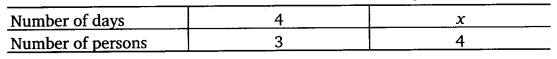Clearly, more is the number of persons, less is the number of days required.
So, it is a case of inverse proportion.
∴ 4 x 3 = x x 4 ⇒ x=$\frac { 4\times 3 }{ 3 }$ = 3
Hence, the job will be completed in 3 days.

Question 7.
A batch of bottles were packed in 25 boxes with 12 bottles in each box. If the same batch is packed using 20 bottles in each box, how many boxes would be filled?

Solution:
Let JC boxes be needed when 20 bottles are packed in each box. Then,Clearly, more is the number of bottles, less will be the number of boxes needed for packing.
So, it is a case of inverse proportion.
∴ 25 x 12 = x x 20 ⇒ x=$\frac { 25\times 12 }{ 20 }$ = 15
Hence, the boxes needed for packing is 15.

Question 8.
A factory requires 42 machines to produce a given number of articles in 63 days. HoW many machines would be required to produce the same number of articles in 54 days?

Solution:
Let x machines are required to produce a fixed number of articles in 54 days. Then,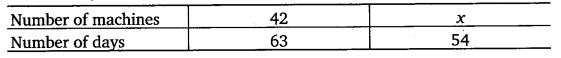Clearly, less is the number of days to produce a fixed number of articles, more machines are required.
So, it is a case of inverse proportion.
∴ 42 x 63 = x x 54 ⇒ x=$\frac { 42\times 63 }{ 54 }$ = 49
Hence, the number of machines needed is 49.

Question 9.
A car takes 2 hours to reach a destination by travelling at the speed of 60 km/h. How long will it take when the car travels at the speed of 80 km/h?

Solution:
Let the car takes x hours to reach a destination by travelling at the speed of 80 km/h. Then,Clearly, more the speed, less will be the time taken. So, it is a case of inverse proportion.
∴ 60 x 2 = 80 x x ⇒ x=$\frac { 60\times 2 }{ 80 }$ =$\frac { 3 }{ 2 }$
Hence, the time taken will be 1$\frac { 1 }{ 2 }$ hours.

Question 10.
Two persons could fit new windows in a house in 3 days.
(i)
One of the persons fell ill before the work started. How long would the job take now?
(ii) How many persons would be needed to fit the windows in one day?

Solution:
(i) Let x days be taken to fix the windows. Then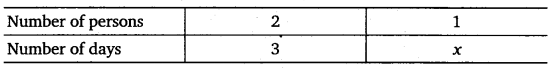Clearly, less the number of persons, more will be the number of days to fix the windows.
So, it is a case of inverse proportion.
∴ 2 x 3 = 1 x x ⇒ x = 6
Hence, one person will finish the given work in 6 days.
(ii) Let x persons will fix the windows in 1 day. Then,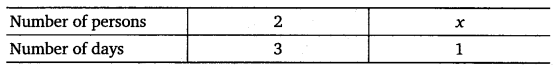Clearly, less the number of days, more will be the number of persons to fix the windows.
So, it is a case of inverse proportion
∴ 2 x 3 = x x 1
⇒ x = 6
Hence, 6 persons will finish the given work in 1 day.

Question 11.
A school has 8 periods a day each of 45 minutes duration. How long would each period he, if the school has 9 periods a day, assuming the number of school hours to be the same?

Solution:
Let x minutes be the duration of period when the school has 9 periods a day. ThenClearly, more the periods, less will be the duration of the period.
So, it is a case of inverse proportion.
∴ 8 x 45 = 9 x x ⇒ x =$\frac { 8\times 45 }{ 9 }$ = 40
Hence, the duration of period is 40 minutes.

### Take Your IIT JEE Coaching to Next Level with Examtube

• Mentoring & Teaching by IITians
• Regular Testing & Analysis
• Preparation for Various Engineering Entrance Exams
• Support for School/Board Exams